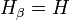# Descendant subgroup

This article defines a subgroup property: a property that can be evaluated to true/false given a group and a subgroup thereof, invariant under subgroup equivalence. View a complete list of subgroup properties[SHOW MORE]
If the ambient group is a finite group, this property is equivalent to the property: subnormal subgroup
View other properties finitarily equivalent to subnormal subgroup | View other variations of subnormal subgroup |
This is a variation of subnormality|Find other variations of subnormality |

## Definition

### Symbol-free definition

A subgroup of a group is said to be descendant if there is a descending series of subgroups indexed by ordinals, each normal in its predecessor, that starts at the whole group, and terminates at the given subgroup.

### Definition with symbols

A subgroup$H$ of a group$G$ is termed descendant if we have a series$H_\alpha$ for every ordinal$\alpha$ such that:

•$H_0 = G$
•$H_{\alpha + 1} \underline{\triangleleft} H_\alpha$ (viz$H_{\alpha + 1}$ is a normal subgroup of$H_\alpha$) for every ordinal$\alpha$.
• If$\alpha$ is a limit ordinal, then$H_\alpha = \bigcap_{\gamma < \alpha} H_\gamma$.

and such that there is some ordinal$\beta$ such that$H_\beta = H$.

### In terms of the descendant closure operator

The subgroup property of being an descendant subgroup is obtained by applying the descendant closure operator to the subgroup property of being normal.

## Facts

### Descendant-contranormal factorization

This result states that given any subgroup$H$ of$G$, there is a unique subgroup$K$ containing$H$ such that$H$ is contranormal in$K$ and$K$ is descendant in$G$.

## Metaproperties

### Transitivity

This subgroup property is transitive: a subgroup with this property in a subgroup with this property, also has this property in the whole group.
ABOUT THIS PROPERTY: View variations of this property that are transitive | View variations of this property that are not transitive
ABOUT TRANSITIVITY: View a complete list of transitive subgroup properties|View a complete list of facts related to transitivity of subgroup properties |Read a survey article on proving transitivity

A descendant subgroup of a descendant subgroup is descendant. The proof relies on simply concatenating the two descending series.

### Trimness

This subgroup property is trim -- it is both trivially true (true for the trivial subgroup) and identity-true (true for a group as a subgroup of itself).
View other trim subgroup properties | View other trivially true subgroup properties | View other identity-true subgroup properties

The trivial subgroup and the whole group are both normal subgroups, hence they are also both descendant subgroups.

### Intermediate subgroup condition

YES: This subgroup property satisfies the intermediate subgroup condition: if a subgroup has the property in the whole group, it has the property in every intermediate subgroup.
ABOUT THIS PROPERTY: View variations of this property satisfying intermediate subgroup condition | View variations of this property not satisfying intermediate subgroup condition
ABOUT INTERMEDIATE SUBROUP CONDITION:View all properties satisfying intermediate subgroup condition | View facts about intermediate subgroup condition

Any descendant subgroup of a group is also descendant in every intermediate subgroup. The proof of this follows by intersecting every member of the descending series with the intermediate subgroup and observing that normality at each stage is preserved.

### Intersection-closedness

YES: This subgroup property is intersection-closed: an arbitrary (nonempty) intersection of subgroups with this property, also has this property.
ABOUT THIS PROPERTY: View variations of this property that are intersection-closed | View variations of this property that are not intersection-closed
ABOUT INTERSECTION-CLOSEDNESS: View all intersection-closed subgroup properties (or, strongly intersection-closed properties) | View all subgroup properties that are not intersection-closed | Read a survey article on proving intersection-closedness | Read a survey article on disproving intersection-closedness

An arbitrary intersection of descendant subgroups is ascendant. This again follows by intersecting the ascending series for each subgroup.